Publication date: 11/10/2021

# Transcendental Functions

You can create a formula that supports transcendental functions, such as logarithmic functions for any base, functions for combinatorial calculations, the Beta function, and several gamma functions. See Transcendental Functions in the Scripting Guide for more information about syntax.

#### Exp

Raises e to the power that you specify. Thus, Exp(1) = e.

#### ExpM1

Returns a more accurate calculation of Exp(x) - 1 when x is very small.

#### Ln

Calculates the natural logarithm of x.

#### Log and Log10

Calculates the natural logarithm (base e). To change the default base, highlight the argument and type a comma or click the Insert key on the Formula Editor keypad. The base appears and is editable. The Log argument can be any numeric expressions. The expression Log(e) evaluates as 1, and Log(32,2) is 5. The Log10 function calculates the logarithm of base 10 only.

#### Log1P

Returns a more accurate calculation of Log(1+x) when x is very small.

#### Squash

Computes the function 1 / (1 + ex), where x is any numeric column, variable, or expression.

#### Logist

Also known as Squish or Logistic, is an efficient computation of the function 1 / (1+e-x), where x is any numeric column, variable, or expression.

#### Root (Square Root)

Calculates the root of its argument as specified by the index. Root initially shows with an index of 2. To change the index, highlight the index argument and enter the value that you want.

#### Factorial

Returns the product of all numbers 1 through the argument that you specify. For example, Factorial(5) evaluates as 120.

#### NChooseK

Returns the number of n things taken k at a time (n select k) and is computed in the standard way using factorials, as n! / (k!(nk)!). For example, NChooseK(5,2) evaluates as 10.

#### Beta

Adds the two parameter Beta function and is written terms of the Gamma function as: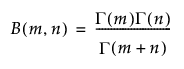#### Gamma

Adds the Gamma function, denoted Γ(i), and is defined as: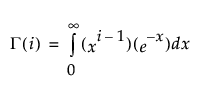Gamma with a single argument is the same as Gamma(x, infinity). The optional second argument changes the upper integer from infinity to the value that you enter. Other interesting gamma function relationships are

for any α > 1, Γ(α) = (α–1) • Γ(α–1)

for any positive integer, n, Γ(n) = (n-1)!

Γ(0.5) = the square root of π

#### LGamma

Is the natural log of the result of the gamma function evaluation. You get the same result using the Log (natural log) function with the Gamma function. However, the LGamma function computes more efficiently than do the Log (natural log) and the Gamma functions together. NChooseK is implemented using LGamma functions. The result is not always an exact integer. If the result is close to an integer, it is rounded up using the Floor function.

#### Digamma

The logarithmic derivative of the Gamma function.

#### Trigamma

The derivative of the Digamma function, or the logarithmic second derivative of the Gamma function.

#### Arrhenius

Calculates the non-specific component of the Arrhenius relationship that is then multiplied by the activation energy in the Arrhenius equation.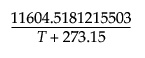#### Arrhenius Inv

The inverse of the Arrhenius function: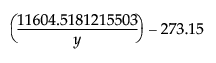#### Logit

Applies the logit transformation to the argument using: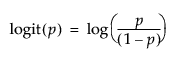#### Logit Percent

Calculates the logit as a percent for the argument.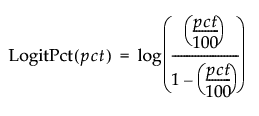#### Logist Percent

Calculates the logistic as a percent for the argument.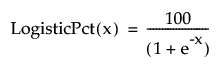#### Scheffe Cubic

Is used in fitting certain models. Scheffe Cubic (X1, X2) is equivalent to X1*X2*(X1-X2).

#### SbInv

Johnson Sb inverse transformation. If argument is normal, the result is Johnson Sb.

#### SbTrans

Johnson Sb transformation from a doubly bound variable to a standard normal (0, 1) distribution.

#### SHASHInv

Returns a transformation of a standard normal variable into a sinh-arcsinh (SHASH) distributed variable.

#### SHASHTrans

Returns a transformation of a sinh-arcsinh (SHASH) distributed variable into a standard normal distributed variable.

#### SlInv

Johnson Sl inverse transformation. If argument is normal, the result is Johnson Sl.

#### SlTrans

Johnson Sl transformation from a doubly bound variable to a standard normal (0, 1) distribution.

#### SuInv

Johnson Su inverse transformation. If argument is normal, the result is Johnson Su.

#### SuTrans

Johnson Su transformation from a doubly bound variable to a standard normal (0, 1) distribution.